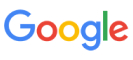New update is available. Click here to update.
Last Updated: 8 Jan, 2021

# Modular Exponentiation

Easy+2 more companies

## Problem statement#### You are given a three integers 'X', 'N', and 'M'. Your task is to find ('X' ^ 'N') % 'M'. A ^ B is defined as A raised to power B and A % C is the remainder when A is divided by C.

##### Input format :
``````The first line of input contains a single integer 'T', representing the number of test cases.

The first line of each test contains three space-separated integers 'X', 'N', and 'M'.
``````
##### Output format :
``````For each test case, return a single line containing the value of ('X' ^ 'N') % 'M'.
``````
##### Note:
``````You don't need to print anything, it has already been taken care of. Just implement the given function.
``````
``````Can you solve the problem in O(log 'N') time complexity and O(1) space complexity?
``````
##### Constraints :
``````1 <= T <= 100
1 <= X, N, M <= 10^9

Time limit: 1 sec
``````## Approaches

### 01 ApproachIn this solution, we will run a loop from 1 to ‘N’ and each time we will multiply ‘X’ to our current product and take modulo of current product with ‘M’.

Make sure to convert variable in long long during multiplication to avoid integer overflow.

Eg.  (10^8 * 10^8) % 10^9 will result in an integer overflow so we will convert it in long long form by multiplying it with 1LL. We need to do (1LL*10^8*10^8)%10^9 to get the correct answer.

The steps are as follows:

1. Declare a variable to store our result, say ‘ANSWER', and initialize it with 1.
2. Run a loop from i = 1 to i = ‘N’, and do:
1. Multiply ‘ANSWER' with ‘X’ and then do modulo with ‘M’ i.e. do ‘ANSWER' = (‘ANSWER' * ‘X’) % ‘M’.